# Math test

In mathematics, there were 25 problems of three kinds: light 2 points, medium 3 points, heavy 5 points, the best score is 84 points. How many points did Jane have when she solved all the easy examples, half medium and one-third difficult?

Correct result:

J =  40

#### Solution: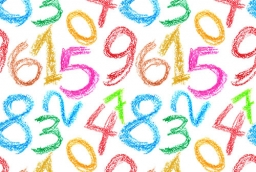We would be pleased if you find an error in the word problem, spelling mistakes, or inaccuracies and send it to us. Thank you!Tips to related online calculators
Do you solve Diofant problems and looking for a calculator of Diofant integer equations?

## Next similar math problems:

• RoundingThe following numbers round to the thousandth:
• Write 2Write 791 thousandths as fraction in expanded form.
• Unknown number 11That number increased by three equals three times itself?
• Eggs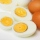One egg boiled in 10 minutes, how long will it take to boil ten eggs at a time?
• SickSick Marcel already taken six tablets, which was a quarter of the total number of pills in the pack. How many pills were in the pack?
• DisjointHow many elements have union and intersection of two disjoint sets when the first have 1 and secodn 8 elements.
• Crocuses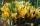The garden grow daffodils, crocuses and roses. 1400 daffodils, crocuses is 462 more and roses is 156 more than crocuses. How many are all the flowers in the garden?
• Perpetrator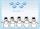The perpetrator is a number that is smaller than the number 80. It is a multiple of five and it is odd. If we added up the tens and ones of this number we get number 8.
• NumberWhat number should be placed instead of the asterisk in number 702*8 to get a number divisible by 6?
• Street numbersLada came to aunt. On the way he noticed that the houses on the left side of the street have odd numbers on the right side and even numbers. The street where he lives aunt, there are 5 houses with an even number, which contains at least one digit number 6
• CagesHonza had three cages (black, silver, gold) and three animals (guinea pig, rat and puppy). There was one animal in each cage. The golden cage stood to the left of the black cage. The silver cage stood on the right of the guinea pig cage. The rat was in th
• Tickets 3A total of 645 tickets were sold for the school play. They were either adult tickets or student tickets. There were 55 fewer student tickets sold than adult tickets. How many adult tickets were sold?
• InfirmaryTwo thirds of children from the infirmary went on a trip seventh went to bathe and 40 children remained in the gym. How many children were treated in the infirmary?
• Ľé sweetsThere are 20 sweets in the bag. Some are chocolate, other coconuts, and the remaining marzipan. Chocolate is 4 times more than coconut. Marzipan's less than chocolate. How much is in a bag of coconut sweets?
• Divisibility by 12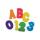Replace the letters A and B by digits so that the resulting number x is divisible by twelve /find all options/. x = 2A3B How many are the overall solutions?
• The math testThe math test contains 20 problems. For each correctly solved problem, the solver gets 3 points, for each incorrectly solved or unsolved problem, 2 points are deducted. Ondrej got 25 points. How many problems did he solve correctly?
• SubjectsStudent makes a mathematical task for five minutes and the task from biology in twelve minutes. Another day he have homework in math and biology which consists of eleven tasks together. To do the job took hour and a half. How many tasks maked in each subj